# Specific requirements

In this programming project, complete the following steps:
• Select a data set and load it into R.
• Apply a survival model to analyze the data of your own choosing.
• Submit a *.html file generated by R Markdown.

# Data frame information

## Name:

Heart Transplant Data

### Description:

The Stanford University Heart Transplant Study was conducted to determine whether an experimental heart transplant program increased lifespan. Each patient entering the program was designated officially a heart transplant candidate, meaning that he was gravely ill and would most likely benefit from a new heart. Then the actual heart transplant occurs between a few weeks to several months depending on the availability of a donor. Very few candidates during this waiting period show improvement and get deselected as a heart transplant candidate, but for the purposes of this experiment those patients were kept in the data as continuing candidates.

#### Usage:

To be used with survival analysis.

#### Format:

A data frame with 103 observations on the following 8 variables.

##### id

ID number of the patient.

##### acceptyear

Year of acceptance as a heart transplant candidate.

##### age

Age of the patient at the beginning of the study.

##### survived

Survival status with levels alive and dead.

##### survtime

Number of days patients were alive after the date they were determined to be a candidate for a heart transplant until the termination date of the study.

##### prior

Whether or not the patient had prior surgery with levels yes and no.

##### transplant

Transplant status with levels control (did not receive a transplant) and treatment (received a transplant).

##### wait

Waiting Time for Transplant.

#### Research Reference:

Turnbull B, Brown B, and Hu M (1974). “Survivorship of heart transplant data.” Journal of the American Statistical Association, vol. 69, pp. 74-80.

# Include the knitr package for integration of R code into Markdown

knitr::opts_chunk$set(echo = TRUE) # Import data library(readr) input_data <- read_csv("stanford.csv", col_types = cols(acceptyear = col_number(), age = col_number(), id = col_number(), prior = col_number(), survived = col_number(), survtime = col_number(), transplant = col_number(), wait = col_number())) # Descriptive statistics ## Dimension of data frame dim(input_data) ##  103 8 ## Structure of data frame str(input_data) ## tibble [103 x 8] (S3: spec_tbl_df/tbl_df/tbl/data.frame) ##$ id        : num [1:103] 15 43 61 75 6 42 54 38 85 2 ...
##  $acceptyear: num [1:103] 68 70 71 72 68 70 71 70 73 68 ... ##$ age       : num [1:103] 53 43 52 52 54 36 47 41 47 51 ...
##  $survived : num [1:103] 1 1 1 1 1 1 1 1 1 1 ... ##$ survtime  : num [1:103] 1 2 2 2 3 3 3 5 5 6 ...
##  $prior : num [1:103] 0 0 0 0 0 0 0 0 0 0 ... ##$ transplant: num [1:103] 0 0 0 0 0 0 0 1 0 0 ...
##  $wait : num [1:103] NA NA NA NA NA NA NA 5 NA NA ... ## - attr(*, "spec")= ## .. cols( ## .. id = col_number(), ## .. acceptyear = col_number(), ## .. age = col_number(), ## .. survived = col_number(), ## .. survtime = col_number(), ## .. prior = col_number(), ## .. transplant = col_number(), ## .. wait = col_number() ## .. ) ## Summary statistics of data frame summary(input_data) ## id acceptyear age survived ## Min. : 1.0 Min. :67.00 Min. : 8.00 Min. :0.0000 ## 1st Qu.: 26.5 1st Qu.:69.00 1st Qu.:41.00 1st Qu.:0.0000 ## Median : 49.0 Median :71.00 Median :47.00 Median :1.0000 ## Mean : 51.4 Mean :70.62 Mean :44.64 Mean :0.7282 ## 3rd Qu.: 77.5 3rd Qu.:72.00 3rd Qu.:52.00 3rd Qu.:1.0000 ## Max. :103.0 Max. :74.00 Max. :64.00 Max. :1.0000 ## ## survtime prior transplant wait ## Min. : 1.0 Min. :0.0000 Min. :0.0000 Min. : 1.00 ## 1st Qu.: 33.5 1st Qu.:0.0000 1st Qu.:0.0000 1st Qu.: 10.00 ## Median : 90.0 Median :0.0000 Median :1.0000 Median : 26.00 ## Mean : 310.2 Mean :0.1165 Mean :0.6699 Mean : 38.42 ## 3rd Qu.: 412.0 3rd Qu.:0.0000 3rd Qu.:1.0000 3rd Qu.: 46.00 ## Max. :1799.0 Max. :1.0000 Max. :1.0000 Max. :310.00 ## NA's :34 ## Glimpse of data frame library(dplyr) ## ## Attaching package: 'dplyr' ## The following objects are masked from 'package:stats': ## ## filter, lag ## The following objects are masked from 'package:base': ## ## intersect, setdiff, setequal, union glimpse(input_data) ## Rows: 103 ## Columns: 8 ##$ id         <dbl> 15, 43, 61, 75, 6, 42, 54, 38, 85, 2, 103, 12, 48, 102, ...
## $acceptyear <dbl> 68, 70, 71, 72, 68, 70, 71, 70, 73, 68, 67, 68, 71, 74, ... ##$ age        <dbl> 53, 43, 52, 52, 54, 36, 47, 41, 47, 51, 39, 53, 56, 40, ...
## $survived <dbl> 1, 1, 1, 1, 1, 1, 1, 1, 1, 1, 1, 1, 1, 0, 1, 1, 1, 1, 1,... ##$ survtime   <dbl> 1, 2, 2, 2, 3, 3, 3, 5, 5, 6, 6, 8, 9, 11, 12, 16, 16, 1...
## $prior <dbl> 0, 0, 0, 0, 0, 0, 0, 0, 0, 0, 0, 0, 0, 0, 0, 0, 0, 0, 0,... ##$ transplant <dbl> 0, 0, 0, 0, 0, 0, 0, 1, 0, 0, 0, 0, 0, 0, 0, 1, 0, 1, 1,...
## $wait <dbl> NA, NA, NA, NA, NA, NA, NA, 5, NA, NA, NA, NA, NA, NA, N... ## Head of data frame head(input_data) ## # A tibble: 6 x 8 ## id acceptyear age survived survtime prior transplant wait ## <dbl> <dbl> <dbl> <dbl> <dbl> <dbl> <dbl> <dbl> ## 1 15 68 53 1 1 0 0 NA ## 2 43 70 43 1 2 0 0 NA ## 3 61 71 52 1 2 0 0 NA ## 4 75 72 52 1 2 0 0 NA ## 5 6 68 54 1 3 0 0 NA ## 6 42 70 36 1 3 0 0 NA ## Tail of data frame tail(input_data) ## # A tibble: 6 x 8 ## id acceptyear age survived survtime prior transplant wait ## <dbl> <dbl> <dbl> <dbl> <dbl> <dbl> <dbl> <dbl> ## 1 14 68 53 1 1386 0 1 37 ## 2 26 69 30 0 1400 0 0 NA ## 3 40 70 48 0 1407 1 1 41 ## 4 34 69 40 0 1571 0 1 23 ## 5 33 69 48 0 1586 0 1 51 ## 6 25 69 33 0 1799 0 1 25 ## Variable data types sapply(input_data,mode) ## id acceptyear age survived survtime prior transplant ## "numeric" "numeric" "numeric" "numeric" "numeric" "numeric" "numeric" ## wait ## "numeric" ## Mean of each variable lapply(input_data,mean) ##$id
##  51.39806
##
## $acceptyear ##  70.62136 ## ##$age
##  44.64078
##
## $survived ##  0.7281553 ## ##$survtime
##  310.1748
##
## $prior ##  0.1165049 ## ##$transplant
##  0.6699029
##
## $wait ##  NA ## Median of each variable lapply(input_data,median) ##$id
##  49
##
## $acceptyear ##  71 ## ##$age
##  47
##
## $survived ##  1 ## ##$survtime
##  90
##
## $prior ##  0 ## ##$transplant
##  1
##
## $wait ##  NA ## Mode of each variable require(modeest) ## Loading required package: modeest lapply(input_data[,c(3,4)],mfv) ##$age
##  47
##
## $survived ##  1 # Variable$Income has no mode. Each value repeats only once.

## Minimum value of each variable

lapply(input_data,min)
## $id ##  1 ## ##$acceptyear
##  67
##
## $age ##  8 ## ##$survived
##  0
##
## $survtime ##  1 ## ##$prior
##  0
##
## $transplant ##  0 ## ##$wait
##  NA

## Maximum value of each variable

lapply(input_data,max)
## $id ##  103 ## ##$acceptyear
##  74
##
## $age ##  64 ## ##$survived
##  1
##
## $survtime ##  1799 ## ##$prior
##  1
##
## $transplant ##  1 ## ##$wait
##  NA

## Range of each variable

lapply(input_data,range)
## $id ##  1 103 ## ##$acceptyear
##  67 74
##
## $age ##  8 64 ## ##$survived
##  0 1
##
## $survtime ##  1 1799 ## ##$prior
##  0 1
##
## $transplant ##  0 1 ## ##$wait
##  NA NA

## Variance of each variable

lapply(input_data,var)
## $id ##  902.3008 ## ##$acceptyear
##  3.610128
##
## $age ##  95.99714 ## ##$survived
##  0.1998858
##
## $survtime ##  183218.5 ## ##$prior
##  0.1039406
##
## $transplant ##  0.223301 ## ##$wait
##  NA

## Standard deviation of each variable

lapply(input_data,sd)
## $id ##  30.03832 ## ##$acceptyear
##  1.900034
##
## $age ##  9.797813 ## ##$survived
##  0.4470859
##
## $survtime ##  428.0403 ## ##$prior
##  0.3223982
##
## $transplant ##  0.4725473 ## ##$wait
##  NA

## Median absolute deviation of each variable

lapply(input_data,mad)
## $id ##  38.5476 ## ##$acceptyear
##  2.9652
##
## $age ##  7.413 ## ##$survived
##  0
##
## $survtime ##  124.5384 ## ##$prior
##  0
##
## $transplant ##  0 ## ##$wait
##  NA

# Survival Analysis

## Data Pre-Processing

### Dichotomous Variable Value Definition

#### survived

1: Alive

#### prior

0: No prior surgery

#### transplant

0: Did Not Receive Heart Transplant

#### age_group

1: Equal to or over 50 years old
0: Younger than 50 years old

### Replacing missing values with zeros

library(tidyr)
library(dplyr)
input_data <- input_data%>% mutate_all(funs(replace_na(.,0)))
## Warning: funs() is soft deprecated as of dplyr 0.8.0
## Please use a list of either functions or lambdas:
##
##   # Simple named list:
##   list(mean = mean, median = median)
##
##   # Auto named with tibble::lst():
##   tibble::lst(mean, median)
##
##   # Using lambdas
##   list(~ mean(., trim = .2), ~ median(., na.rm = TRUE))
## This warning is displayed once per session.
head(input_data,n=25)
## # A tibble: 25 x 8
##       id acceptyear   age survived survtime prior transplant  wait
##    <dbl>      <dbl> <dbl>    <dbl>    <dbl> <dbl>      <dbl> <dbl>
##  1    15         68    53        1        1     0          0     0
##  2    43         70    43        1        2     0          0     0
##  3    61         71    52        1        2     0          0     0
##  4    75         72    52        1        2     0          0     0
##  5     6         68    54        1        3     0          0     0
##  6    42         70    36        1        3     0          0     0
##  7    54         71    47        1        3     0          0     0
##  8    38         70    41        1        5     0          1     5
##  9    85         73    47        1        5     0          0     0
## 10     2         68    51        1        6     0          0     0
## # ... with 15 more rows

### Dichotomize the variable age

input_data <- input_data %>% mutate(age_group = ifelse(age >=50, 1, 0))
input_data$age_group <- factor(input_data$age_group)
head(input_data, n=25)
## # A tibble: 25 x 9
##       id acceptyear   age survived survtime prior transplant  wait age_group
##    <dbl>      <dbl> <dbl>    <dbl>    <dbl> <dbl>      <dbl> <dbl> <fct>
##  1    15         68    53        1        1     0          0     0 1
##  2    43         70    43        1        2     0          0     0 0
##  3    61         71    52        1        2     0          0     0 1
##  4    75         72    52        1        2     0          0     0 1
##  5     6         68    54        1        3     0          0     0 1
##  6    42         70    36        1        3     0          0     0 0
##  7    54         71    47        1        3     0          0     0 0
##  8    38         70    41        1        5     0          1     5 0
##  9    85         73    47        1        5     0          0     0 0
## 10     2         68    51        1        6     0          0     0 1
## # ... with 15 more rows

## Summary of dependent variables

summary(input_data$survtime) ## Min. 1st Qu. Median Mean 3rd Qu. Max. ## 1.0 33.5 90.0 310.2 412.0 1799.0 summary(input_data$survived)
##    Min. 1st Qu.  Median    Mean 3rd Qu.    Max.
##  0.0000  0.0000  1.0000  0.7282  1.0000  1.0000

## Summary of independent variables

summary(cbind(acceptyear=input_data$acceptyear,age=input_data$age,prior=input_data$prior,wait=input_data$wait))
##    acceptyear         age            prior             wait
##  Min.   :67.00   Min.   : 8.00   Min.   :0.0000   Min.   :  0.00
##  1st Qu.:69.00   1st Qu.:41.00   1st Qu.:0.0000   1st Qu.:  0.00
##  Median :71.00   Median :47.00   Median :0.0000   Median : 10.00
##  Mean   :70.62   Mean   :44.64   Mean   :0.1165   Mean   : 25.74
##  3rd Qu.:72.00   3rd Qu.:52.00   3rd Qu.:0.0000   3rd Qu.: 32.00
##  Max.   :74.00   Max.   :64.00   Max.   :1.0000   Max.   :310.00

## Summary of group variables

summary(input_data$transpant) ## Warning: Unknown or uninitialised column: transpant. ## Length Class Mode ## 0 NULL NULL summary(input_data$age)
##    Min. 1st Qu.  Median    Mean 3rd Qu.    Max.
##    8.00   41.00   47.00   44.64   52.00   64.00

## Kaplan-Meier Survival Analysis

### Explanation

Kaplan-Meier estimator is a non-parametric statistic used for estimating the survival probability of certain events. The estimation is based on the survival duration of such an event and other factors such as grouping and treatment. By non-parametric, it means that it is not a regression method where the magnitude and direction of the relationship between dependent variable and independent variables are determined by the coefficients. In other words, this method has no control for confounders through the coefficients.

### Assumption

• patient s and events are independent of each other
• Censoring does not affect the survival probability

### Kaplan-Meier Survival Analysis (Group by Prior Surgery)

#### Observation

patient s who had prior surgeries have a higher probability of surviving. 50% of patient s with prior surgeries survived up to about 990 days. However, 50% of patient s with no prior surgery survived up to only about 100 days.

library(survival)
km.md <- survfit(Surv(survtime,survived) ~ prior, data = input_data)
km.md
## Call: survfit(formula = Surv(survtime, survived) ~ prior, data = input_data)
##
##          n events median 0.95LCL 0.95UCL
## prior=0 91     69     78      61     153
## prior=1 12      6    979     583      NA
summary(km.md)
## Call: survfit(formula = Surv(survtime, survived) ~ prior, data = input_data)
##
##                 prior=0
##  time n.risk n.event survival std.err lower 95% CI upper 95% CI
##     1     91       1    0.989  0.0109       0.9678        1.000
##     2     90       3    0.956  0.0215       0.9148        0.999
##     3     87       3    0.923  0.0279       0.8699        0.979
##     5     84       2    0.901  0.0313       0.8418        0.965
##     6     82       2    0.879  0.0342       0.8146        0.949
##     8     80       1    0.868  0.0355       0.8013        0.941
##     9     79       1    0.857  0.0367       0.7882        0.932
##    12     77       1    0.846  0.0379       0.7750        0.924
##    16     76       3    0.813  0.0410       0.7361        0.897
##    17     73       1    0.801  0.0419       0.7234        0.888
##    18     72       1    0.790  0.0428       0.7108        0.879
##    21     71       2    0.768  0.0444       0.6859        0.860
##    28     69       1    0.757  0.0451       0.6735        0.851
##    30     68       1    0.746  0.0458       0.6613        0.841
##    32     66       1    0.735  0.0465       0.6489        0.831
##    35     65       1    0.723  0.0471       0.6365        0.822
##    36     64       1    0.712  0.0477       0.6243        0.812
##    37     63       1    0.701  0.0483       0.6121        0.802
##    39     62       1    0.689  0.0488       0.6000        0.792
##    40     61       2    0.667  0.0497       0.5760        0.772
##    43     59       1    0.655  0.0502       0.5641        0.762
##    45     58       1    0.644  0.0506       0.5523        0.751
##    50     57       1    0.633  0.0509       0.5405        0.741
##    51     56       1    0.622  0.0513       0.5288        0.731
##    53     55       1    0.610  0.0516       0.5171        0.720
##    58     54       1    0.599  0.0518       0.5055        0.710
##    61     53       1    0.588  0.0521       0.4940        0.699
##    66     52       1    0.576  0.0523       0.4825        0.688
##    68     51       2    0.554  0.0526       0.4596        0.667
##    69     49       1    0.542  0.0527       0.4483        0.656
##    72     48       2    0.520  0.0529       0.4258        0.635
##    77     46       1    0.509  0.0529       0.4146        0.624
##    78     45       1    0.497  0.0530       0.4035        0.613
##    80     44       1    0.486  0.0529       0.3925        0.602
##    81     43       1    0.475  0.0529       0.3815        0.591
##    85     42       1    0.463  0.0528       0.3705        0.579
##    90     41       1    0.452  0.0527       0.3596        0.568
##    96     40       1    0.441  0.0526       0.3487        0.557
##   100     39       1    0.429  0.0525       0.3380        0.546
##   102     38       1    0.418  0.0523       0.3272        0.534
##   110     36       1    0.407  0.0521       0.3162        0.523
##   149     34       1    0.395  0.0519       0.3048        0.511
##   153     33       1    0.383  0.0517       0.2935        0.499
##   188     31       1    0.370  0.0515       0.2819        0.486
##   207     30       1    0.358  0.0512       0.2703        0.474
##   219     29       1    0.346  0.0509       0.2588        0.461
##   263     28       1    0.333  0.0506       0.2474        0.449
##   285     26       2    0.308  0.0498       0.2239        0.423
##   308     24       1    0.295  0.0494       0.2123        0.409
##   334     23       1    0.282  0.0489       0.2007        0.396
##   340     22       1    0.269  0.0483       0.1893        0.383
##   675     12       1    0.247  0.0492       0.1669        0.365
##   733     11       1    0.224  0.0496       0.1454        0.346
##   852      9       1    0.199  0.0499       0.1220        0.326
##  1032      6       1    0.166  0.0515       0.0905        0.305
##  1386      5       1    0.133  0.0508       0.0628        0.281
##
##                 prior=1
##  time n.risk n.event survival std.err lower 95% CI upper 95% CI
##   165     11       1    0.909  0.0867        0.754        1.000
##   186     10       1    0.818  0.1163        0.619        1.000
##   342      9       1    0.727  0.1343        0.506        1.000
##   583      6       1    0.606  0.1574        0.364        1.000
##   979      5       1    0.485  0.1661        0.248        0.949
##   995      4       1    0.364  0.1629        0.151        0.875
plot(km.md, conf.int=T, xlab="Survival Time (days)", ylab="Survival Probability (%)", main="Kaplan-Meier Model (Group by Prior Surgery)",las=1, lty=1, lwd=2, mark.time=TRUE, col=c("green","blue"))
abline(h=0.5,col="red")
legend(1000,0.80, legend = c("No Prior Surgery","Had Prior Surgery","50% Survival Probability Line"), lty=1, lwd=2,col=c("green", "blue", "red"), bty="", cex=0.6)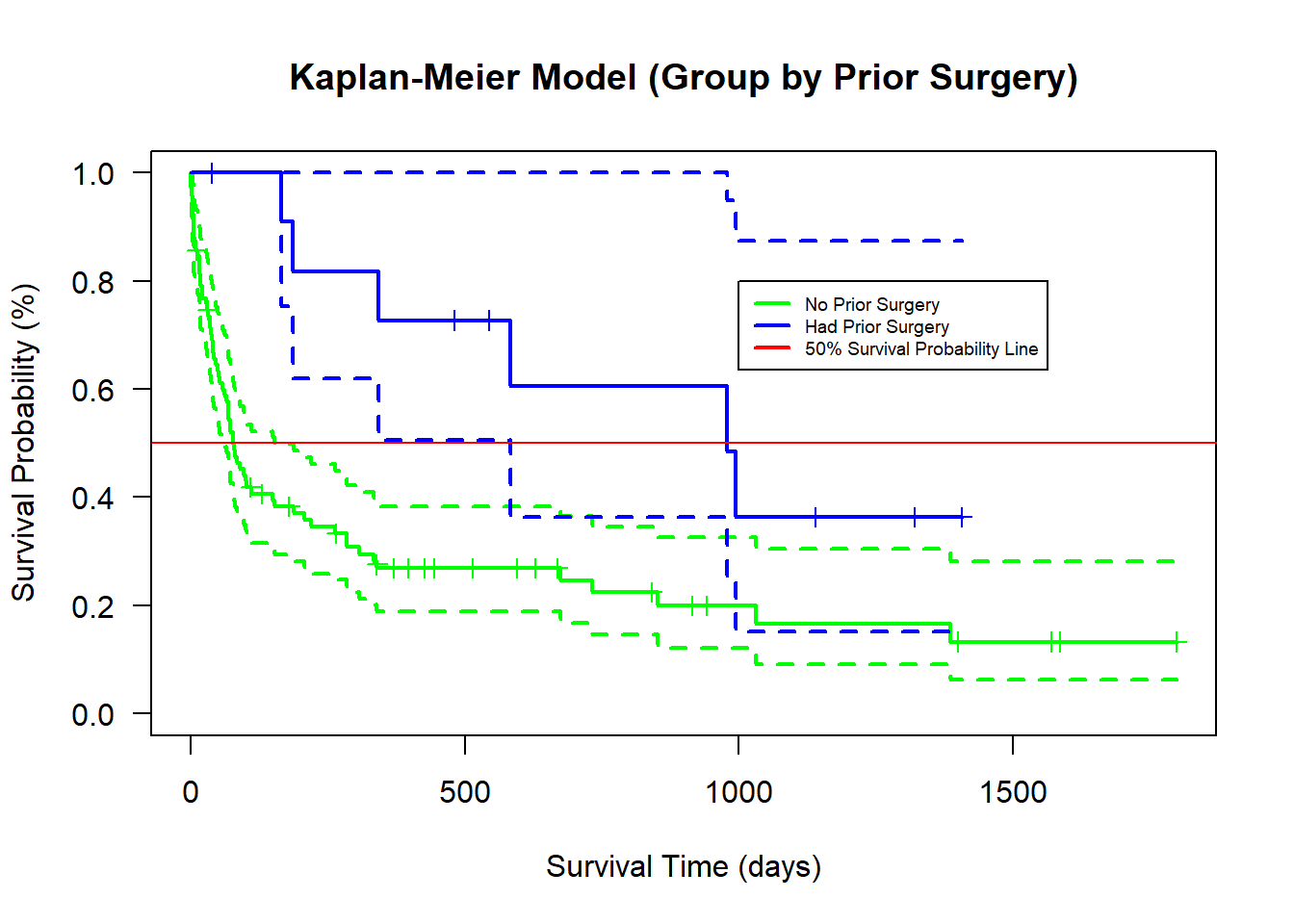#### Log-Rank Test

##### Ho

No difference in survival S(t) between the two groups

##### Ha

There is difference in survival S(t) between the two groups

##### Observation

Given an alpha or cut-off point of 0.05, a p-value of 0.01 indicates the difference between the two survival groups is highly significant. Therefore, the null hypothesis Ho is rejected and the alternative hypothesis Ha is accepted.

library(survival)
surv.diff <- survdiff(Surv(survtime,survived) ~ prior, data = input_data)
surv.diff
## Call:
## survdiff(formula = Surv(survtime, survived) ~ prior, data = input_data)
##
##          N Observed Expected (O-E)^2/E (O-E)^2/V
## prior=0 91       69     60.3      1.24      6.59
## prior=1 12        6     14.7      5.11      6.59
##
##  Chisq= 6.6  on 1 degrees of freedom, p= 0.01

### Kaplan-Meier Survival Analysis (Group by Transplant)

#### Observation

patient s who did receive the heart transplant have a higher probability of surviving. 50% of patient s who did receive the heart transplant survived up to about 300 days. However, 50% of patient s who did not receive the heart transplant survived up to only about 10 days.

library(survival)
library(survminer)
## Loading required package: ggplot2
## Loading required package: ggpubr
## Loading required package: magrittr
##
## Attaching package: 'magrittr'
## The following object is masked from 'package:tidyr':
##
##     extract
km.md <- survfit(Surv(survtime,survived) ~ transplant, data = input_data)
km.md
## Call: survfit(formula = Surv(survtime, survived) ~ transplant, data = input_data)
##
##               n events median 0.95LCL 0.95UCL
## transplant=0 34     30     21      12      50
## transplant=1 69     45    285     153     852
summary(km.md)
## Call: survfit(formula = Surv(survtime, survived) ~ transplant, data = input_data)
##
##                 transplant=0
##  time n.risk n.event survival std.err lower 95% CI upper 95% CI
##     1     34       1   0.9706  0.0290       0.9154        1.000
##     2     33       3   0.8824  0.0553       0.7804        0.998
##     3     30       3   0.7941  0.0693       0.6692        0.942
##     5     27       1   0.7647  0.0727       0.6346        0.921
##     6     26       2   0.7059  0.0781       0.5682        0.877
##     8     24       1   0.6765  0.0802       0.5362        0.853
##     9     23       1   0.6471  0.0820       0.5048        0.829
##    12     21       1   0.6162  0.0836       0.4723        0.804
##    16     20       1   0.5854  0.0849       0.4405        0.778
##    18     19       1   0.5546  0.0859       0.4094        0.751
##    21     18       2   0.4930  0.0867       0.3493        0.696
##    32     15       1   0.4601  0.0869       0.3177        0.666
##    35     14       1   0.4273  0.0867       0.2871        0.636
##    36     13       1   0.3944  0.0860       0.2572        0.605
##    37     12       1   0.3615  0.0849       0.2281        0.573
##    40     11       2   0.2958  0.0812       0.1727        0.507
##    50      9       1   0.2629  0.0786       0.1464        0.472
##    69      8       1   0.2301  0.0753       0.1211        0.437
##    85      7       1   0.1972  0.0714       0.0970        0.401
##   102      6       1   0.1643  0.0666       0.0743        0.364
##   149      5       1   0.1315  0.0609       0.0531        0.326
##   263      4       1   0.0986  0.0538       0.0338        0.287
##   340      3       1   0.0657  0.0448       0.0173        0.250
##
##                 transplant=1
##  time n.risk n.event survival std.err lower 95% CI upper 95% CI
##     5     69       1    0.986  0.0144       0.9577        1.000
##    16     68       2    0.957  0.0246       0.9096        1.000
##    17     66       1    0.942  0.0281       0.8885        0.999
##    28     65       1    0.928  0.0312       0.8683        0.991
##    30     64       1    0.913  0.0339       0.8489        0.982
##    39     63       1    0.899  0.0363       0.8301        0.973
##    43     61       1    0.884  0.0386       0.8113        0.963
##    45     60       1    0.869  0.0407       0.7929        0.953
##    51     59       1    0.854  0.0426       0.7748        0.942
##    53     58       1    0.840  0.0443       0.7571        0.931
##    58     57       1    0.825  0.0459       0.7396        0.920
##    61     56       1    0.810  0.0474       0.7224        0.909
##    66     55       1    0.795  0.0488       0.7053        0.897
##    68     54       2    0.766  0.0512       0.6719        0.873
##    72     52       2    0.737  0.0533       0.6391        0.849
##    77     50       1    0.722  0.0543       0.6229        0.836
##    78     49       1    0.707  0.0551       0.6069        0.824
##    80     48       1    0.692  0.0559       0.5910        0.811
##    81     47       1    0.678  0.0566       0.5752        0.798
##    90     46       1    0.663  0.0573       0.5596        0.785
##    96     45       1    0.648  0.0579       0.5441        0.772
##   100     44       1    0.633  0.0584       0.5287        0.759
##   110     42       1    0.618  0.0589       0.5130        0.745
##   153     40       1    0.603  0.0594       0.4969        0.731
##   165     39       1    0.587  0.0599       0.4810        0.717
##   186     37       1    0.572  0.0603       0.4647        0.703
##   188     36       1    0.556  0.0607       0.4485        0.688
##   207     35       1    0.540  0.0610       0.4325        0.674
##   219     34       1    0.524  0.0613       0.4166        0.659
##   285     32       2    0.491  0.0616       0.3840        0.628
##   308     30       1    0.475  0.0617       0.3680        0.613
##   334     29       1    0.458  0.0617       0.3521        0.597
##   342     27       1    0.441  0.0617       0.3356        0.581
##   583     20       1    0.419  0.0625       0.3132        0.562
##   675     16       1    0.393  0.0638       0.2860        0.540
##   733     15       1    0.367  0.0647       0.2597        0.519
##   852     13       1    0.339  0.0656       0.2317        0.495
##   979     10       1    0.305  0.0672       0.1979        0.470
##   995      9       1    0.271  0.0678       0.1660        0.442
##  1032      8       1    0.237  0.0672       0.1360        0.413
##  1386      5       1    0.190  0.0685       0.0935        0.385
plot(km.md, conf.int=T, xlab="Survival Time (days)", ylab="Survival Probability (%)", main="Kaplan-Meier Model (Group by Transplant)",las=1, lty=1, lwd=2, mark.time=TRUE, col=c("green","blue"))
abline(h=0.5,col="red")
legend(800,0.95, legend = c("Did Not Receive Heart Transplant","Did Receive Heart Transplant","50% Survival Probability Line"), lty=1, lwd=2,col=c("green", "blue", "red"), bty="", cex=0.6)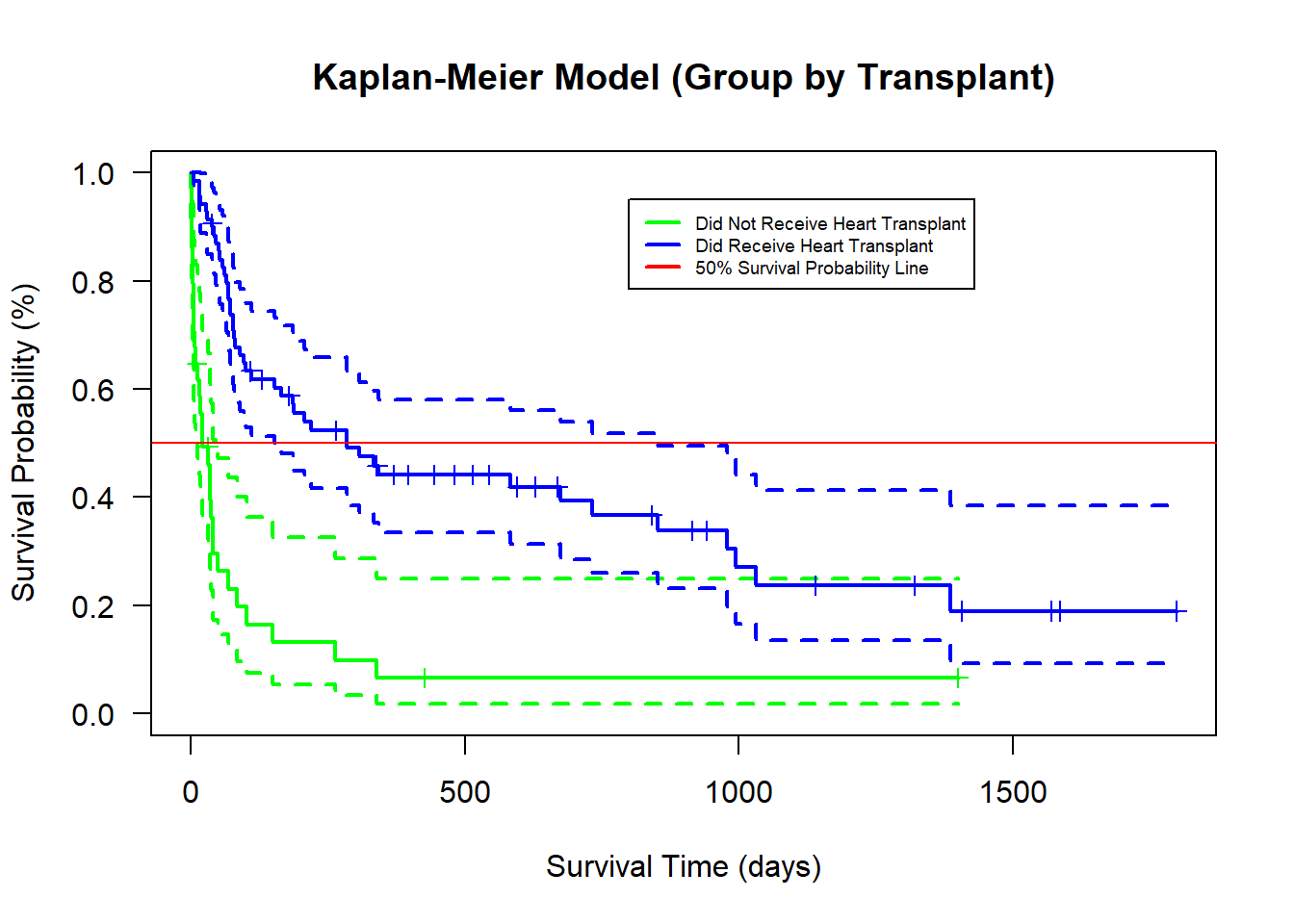#### Log-Rank Test

##### Ho

No difference in survival S(t) between the two groups

##### Ha

There is difference in survival S(t) between the two groups

##### Observation

Given an alpha or cut-off point of 0.05, a p-value of 8e-09 indicates the difference between the two survival groups is highly significant. Therefore, the null hypothesis Ho is rejected and the alternative hypothesis Ha is accepted.

library(survival)
surv.diff <- survdiff(Surv(survtime,survived) ~ transplant, data = input_data)
surv.diff
## Call:
## survdiff(formula = Surv(survtime, survived) ~ transplant, data = input_data)
##
##               N Observed Expected (O-E)^2/E (O-E)^2/V
## transplant=0 34       30     12.1      26.5      33.2
## transplant=1 69       45     62.9       5.1      33.2
##
##  Chisq= 33.2  on 1 degrees of freedom, p= 8e-09

### Kaplan-Meier Survival Analysis (Group by Age)

#### Observation

patient s who were younger than 50 years old have a higher probability of surviving. 50% of patient s who were younger than 50 years old survived up to about 250 days. However, 50% of patient s who were equal to or older than 50 years old survived up to only about 20 days.

library(survival)
library(survminer)
km.md <- survfit(Surv(survtime,survived) ~ age_group, data = input_data)
km.md
## Call: survfit(formula = Surv(survtime, survived) ~ age_group, data = input_data)
##
##              n events median 0.95LCL 0.95UCL
## age_group=0 69     44  263.0     102     852
## age_group=1 34     31   63.5      35      90
summary(km.md)
## Call: survfit(formula = Surv(survtime, survived) ~ age_group, data = input_data)
##
##                 age_group=0
##  time n.risk n.event survival std.err lower 95% CI upper 95% CI
##     2     69       1    0.986  0.0144        0.958        1.000
##     3     68       2    0.957  0.0246        0.910        1.000
##     5     66       2    0.928  0.0312        0.868        0.991
##     6     64       1    0.913  0.0339        0.849        0.982
##    12     62       1    0.898  0.0364        0.830        0.973
##    16     61       1    0.884  0.0387        0.811        0.963
##    17     60       1    0.869  0.0408        0.793        0.953
##    18     59       1    0.854  0.0426        0.775        0.942
##    21     58       2    0.825  0.0460        0.739        0.920
##    36     55       1    0.810  0.0475        0.722        0.908
##    39     54       1    0.795  0.0490        0.704        0.897
##    40     52       2    0.764  0.0516        0.669        0.872
##    45     50       1    0.749  0.0528        0.652        0.860
##    50     49       1    0.734  0.0539        0.635        0.847
##    51     48       1    0.718  0.0549        0.618        0.834
##    58     47       1    0.703  0.0558        0.602        0.821
##    68     46       2    0.672  0.0574        0.569        0.795
##    69     44       1    0.657  0.0581        0.553        0.782
##    72     43       1    0.642  0.0587        0.536        0.768
##    85     42       1    0.627  0.0593        0.521        0.754
##   100     41       1    0.611  0.0598        0.505        0.740
##   102     40       1    0.596  0.0602        0.489        0.727
##   110     38       1    0.580  0.0606        0.473        0.712
##   149     36       1    0.564  0.0611        0.456        0.698
##   153     35       1    0.548  0.0614        0.440        0.683
##   165     34       1    0.532  0.0617        0.424        0.668
##   188     32       1    0.515  0.0619        0.407        0.652
##   263     31       1    0.499  0.0621        0.391        0.637
##   285     29       2    0.464  0.0624        0.357        0.604
##   308     27       1    0.447  0.0624        0.340        0.588
##   334     26       1    0.430  0.0624        0.324        0.571
##   340     25       1    0.413  0.0622        0.307        0.555
##   342     23       1    0.395  0.0620        0.290        0.537
##   583     18       1    0.373  0.0623        0.269        0.517
##   852     13       1    0.344  0.0638        0.239        0.495
##   979     10       1    0.310  0.0661        0.204        0.470
##   995      9       1    0.275  0.0671        0.171        0.444
##  1032      8       1    0.241  0.0669        0.140        0.415
##
##                 age_group=1
##  time n.risk n.event survival std.err lower 95% CI upper 95% CI
##     1     34       1   0.9706  0.0290       0.9154        1.000
##     2     33       2   0.9118  0.0486       0.8212        1.000
##     3     31       1   0.8824  0.0553       0.7804        0.998
##     6     30       1   0.8529  0.0607       0.7418        0.981
##     8     29       1   0.8235  0.0654       0.7049        0.962
##     9     28       1   0.7941  0.0693       0.6692        0.942
##    16     27       2   0.7353  0.0757       0.6010        0.900
##    28     25       1   0.7059  0.0781       0.5682        0.877
##    30     24       1   0.6765  0.0802       0.5362        0.853
##    32     23       1   0.6471  0.0820       0.5048        0.829
##    35     22       1   0.6176  0.0833       0.4741        0.805
##    37     21       1   0.5882  0.0844       0.4440        0.779
##    43     20       1   0.5588  0.0852       0.4145        0.753
##    53     19       1   0.5294  0.0856       0.3856        0.727
##    61     18       1   0.5000  0.0857       0.3573        0.700
##    66     17       1   0.4706  0.0856       0.3295        0.672
##    72     16       1   0.4412  0.0852       0.3022        0.644
##    77     15       1   0.4118  0.0844       0.2755        0.615
##    78     14       1   0.3824  0.0833       0.2494        0.586
##    80     13       1   0.3529  0.0820       0.2239        0.556
##    81     12       1   0.3235  0.0802       0.1990        0.526
##    90     11       1   0.2941  0.0781       0.1747        0.495
##    96     10       1   0.2647  0.0757       0.1512        0.464
##   186      9       1   0.2353  0.0727       0.1284        0.431
##   207      8       1   0.2059  0.0693       0.1064        0.398
##   219      7       1   0.1765  0.0654       0.0854        0.365
##   675      3       1   0.1176  0.0649       0.0399        0.347
##   733      2       1   0.0588  0.0527       0.0101        0.341
##  1386      1       1   0.0000     NaN           NA           NA
plot(km.md, conf.int=T, xlab="Survival Time (days)", ylab="Survival Probability (%)", main="Kaplan-Meier Model (Group by Age)",las=1, lty=1, lwd=2, mark.time=TRUE, col=c("green","blue"))
abline(h=0.5,col="red")
legend(800,0.95, legend = c("Younger than 50 years old","Equal to or over 50 years old","50% Survival Probability Line"), lty=1, lwd=2,col=c("green", "blue", "red"), bty="", cex=0.6)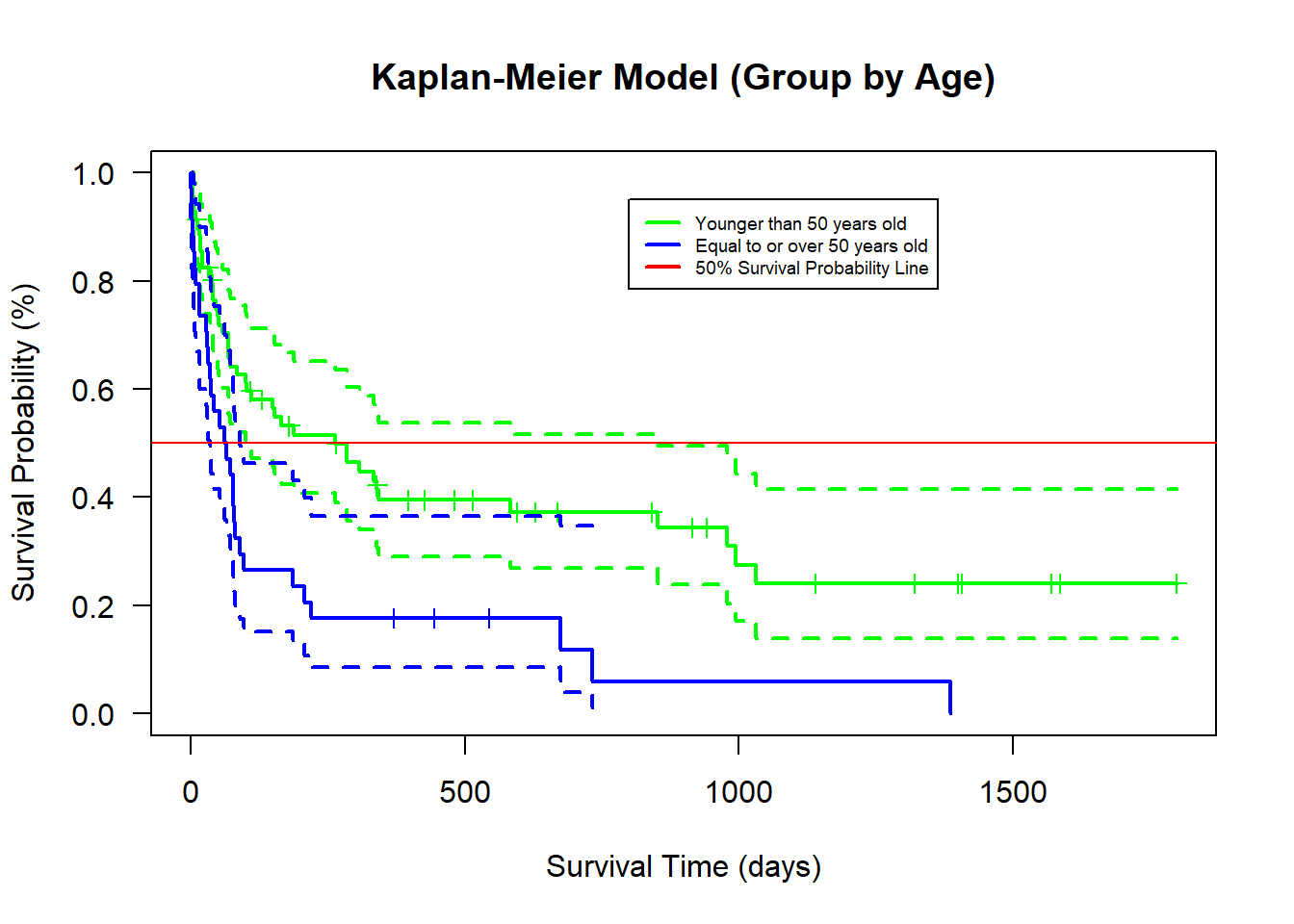#### Log-Rank Test

##### Ho

No difference in survival S(t) between the two groups

##### Ha

There is difference in survival S(t) between the two groups

##### Observation

Given an alpha or cut-off point of 0.05, a p-value of 7e-04 indicates a high distinction between the two survival groups. Therefore, the null hypothesis Ho is rejected and the alternative hypothesis Ha is accepted.

library(survival)
surv.diff <- survdiff(Surv(survtime,survived) ~ age_group, data = input_data)
surv.diff
## Call:
## survdiff(formula = Surv(survtime, survived) ~ age_group, data = input_data)
##
##              N Observed Expected (O-E)^2/E (O-E)^2/V
## age_group=0 69       44     56.5      2.76      11.5
## age_group=1 34       31     18.5      8.44      11.5
##
##  Chisq= 11.5  on 1 degrees of freedom, p= 7e-04

## Cox Proportional-Hazards Survival Analysis

### Explanation

The Cox proportional-hazards method is a semi-parametric regression model used for estimating the association between the survival time of certain patient s and one or more independent variables. By semi-parametric, it means that it is a regression method where the magnitude and direction of the relationship between the dependent variable and independent variables could be determined by the coefficients. In other words, this method has control for confounders through the coefficients.

### Assumption

• Log Hazard Linearity (Log hazard ratio ln(h(t)) is a function of the independent x variables)
• Proportiona Hazard (Hazard ratio h(t) or relative difference between groups remain constant over time)
• Baseline hazard is unspecified
• X-values do not change over time
• Survival curves do not cross
• Patients and events are independent of each other
• Censoring does not affect the survival probability

### Regression Iteration

#### Observation

Both of the independent variables prior and wait have p-values of 1, which indicates a lack of significant relationship with the dependent variable (survtime, survived). However, the independent variable transplant has a p-value of 0.0, which indicates a very high significant relationship with the dependent variable. Independent variable age and dummy variable age_group1 have a moderately significant relationship with the dependent variable. Given a range between 0 and 1, a concordance value of 0.762 indicates a very good fitted model. In other words, the direction of the predicted relative survival probabilities for a pair of patients is the same as that of the actual observed relative survival probabilities. The likelihood ratio test, Wald test, and Log Rank test are used for testing whether the null hypothesis that all coefficients in the model are zeros. Since none of the p-values is greater than 0.05, the null hypothesis is rejected. Therefore, the alternative hypothesis that at least one of the coefficients is not equal to zero is accepted.

library(survival)
cox.md <- coxph(Surv(survtime,survived) ~ prior+transplant+age_group+age+wait, data = input_data)
summary(cox.md)
## Call:
## coxph(formula = Surv(survtime, survived) ~ prior + transplant +
##     age_group + age + wait, data = input_data)
##
##   n= 103, number of events= 75
##
##                 coef exp(coef)  se(coef)      z Pr(>|z|)
## prior      -0.506746  0.602453  0.456183 -1.111   0.2666
## transplant -1.435108  0.238090  0.318497 -4.506 6.61e-06 ***
## age_group1  0.678363  1.970649  0.339777  1.996   0.0459 *
## age         0.034545  1.035148  0.017540  1.969   0.0489 *
## wait       -0.008285  0.991749  0.005057 -1.639   0.1013
## ---
## Signif. codes:  0 '***' 0.001 '**' 0.01 '*' 0.05 '.' 0.1 ' ' 1
##
##            exp(coef) exp(-coef) lower .95 upper .95
## prior         0.6025     1.6599    0.2464    1.4731
## transplant    0.2381     4.2001    0.1275    0.4445
## age_group1    1.9706     0.5074    1.0125    3.8356
## age           1.0351     0.9660    1.0002    1.0714
## wait          0.9917     1.0083    0.9820    1.0016
##
## Concordance= 0.762  (se = 0.028 )
## Likelihood ratio test= 55.41  on 5 df,   p=1e-10
## Wald test            = 51.7  on 5 df,   p=6e-10
## Score (logrank) test = 60.45  on 5 df,   p=1e-11

### Log Hazard Linearity Test

#### Observation

Both the “martingale” plot and the “deviance” plot clearly indicate the existence of linearity relationship between the Log hazard ratio ln(h(t)) and the independent x variables.

library(survival)
cox.md <- coxph(Surv(survtime,survived) ~ prior+transplant+age_group+age+wait, data = input_data)

plot(predict(cox.md),residuals(cox.md,type="martingale"),
xlab="Fitted Values",
ylab="Martingale Residuals",
main="Residual Plot",
las=1
)
abline(h=0,col=2)

lines(smooth.spline(predict(cox.md),residuals(cox.md,type="martingale")),col="blue")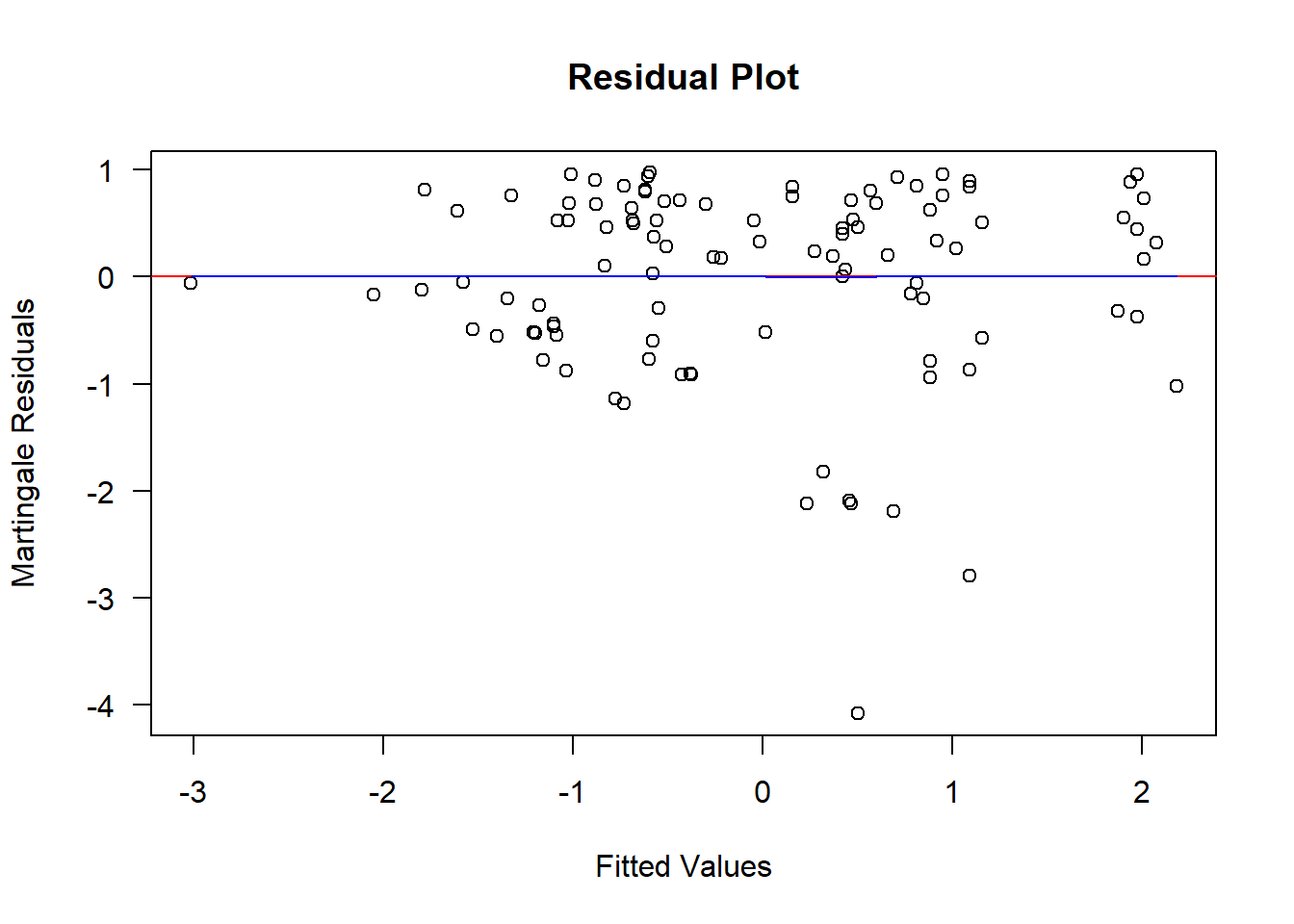plot(predict(cox.md),residuals(cox.md,type="deviance"),
xlab="Fitted Values",
ylab="Deviance Residuals",
main="Residual Plot",
las=1
)
abline(h=0,col=2)

lines(smooth.spline(predict(cox.md),residuals(cox.md,type="deviance")),col="blue")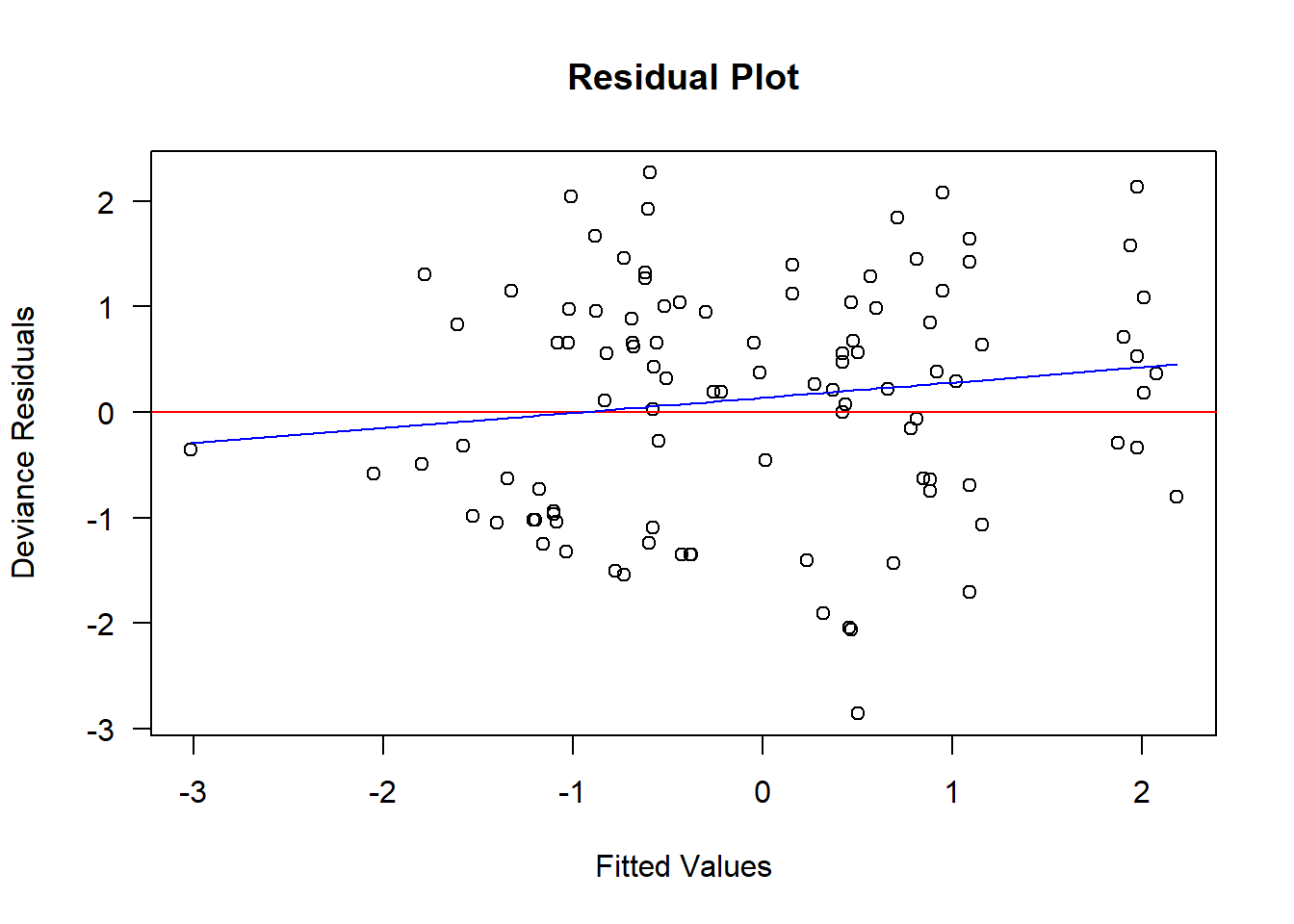### Schoenfeld’s Proportional Hazard Ratio Test

#### Observation

The only two plots that seem to pass the Proportional Hazard Ratio test are the plots of Age versus Time and Age_group versus Time. The rest of the plots raise suspicion that the proportional hazard assumption is very likely to be violated.

library(survival)
cox.md <- coxph(Surv(survtime,survived) ~ prior+transplant+age_group+age+wait, data = input_data)
par(mfrom=c(1,1))
## Warning in par(mfrom = c(1, 1)): "mfrom" is not a graphical parameter
plot(cox.zph(cox.md))
abline(h=0,col=2)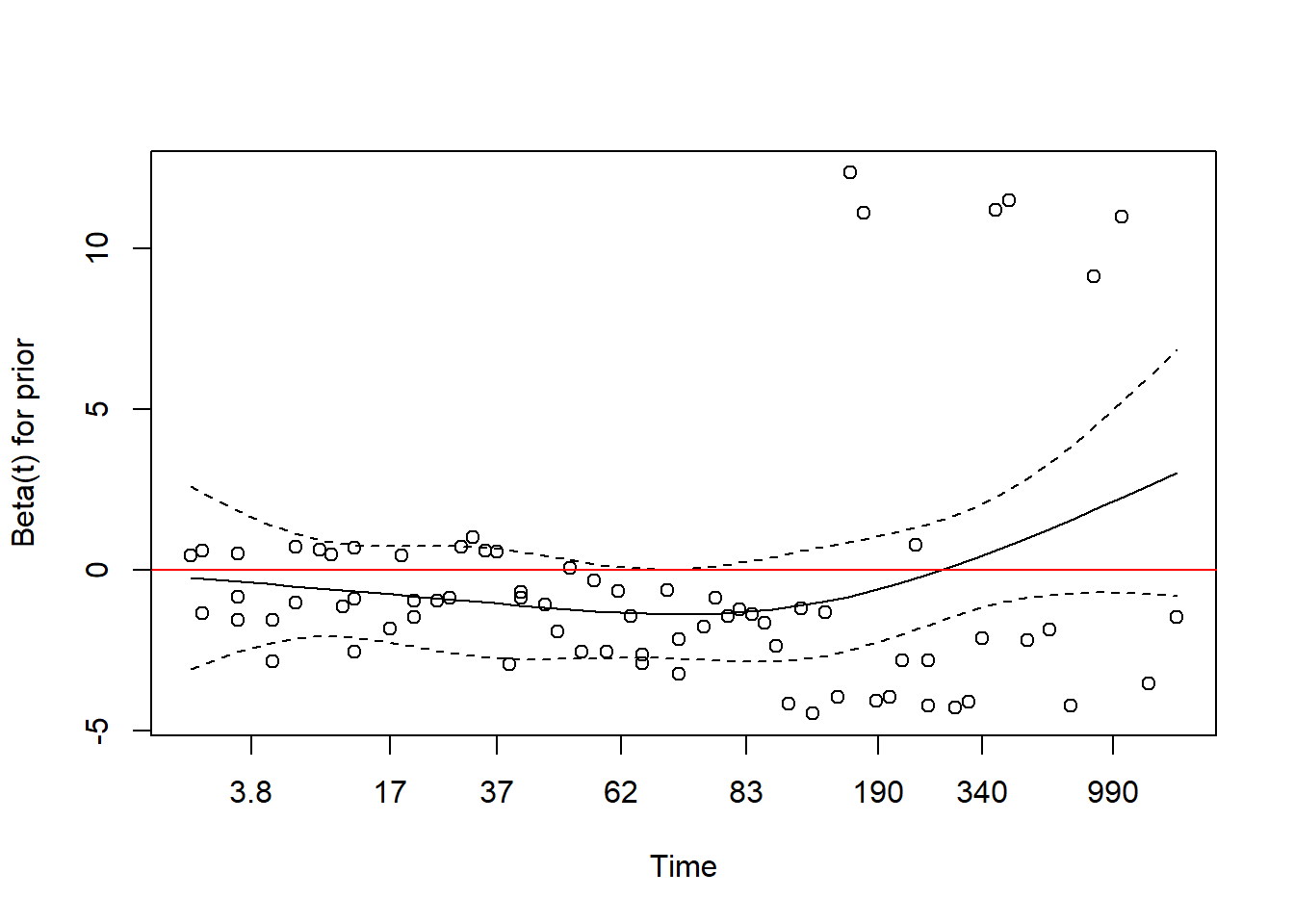plot(cox.zph(cox.md))
abline(h=0,col=2)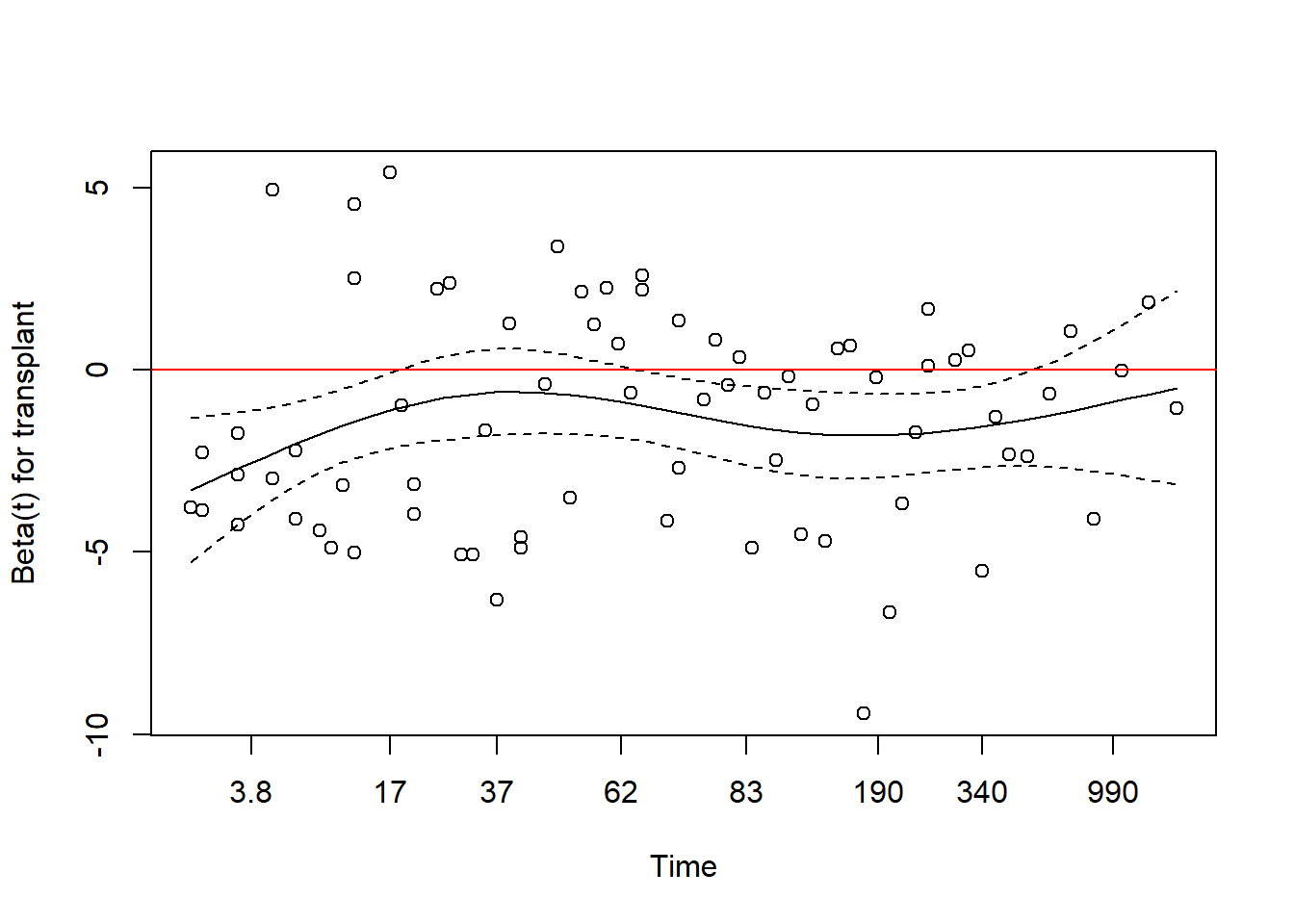plot(cox.zph(cox.md))
abline(h=0,col=2)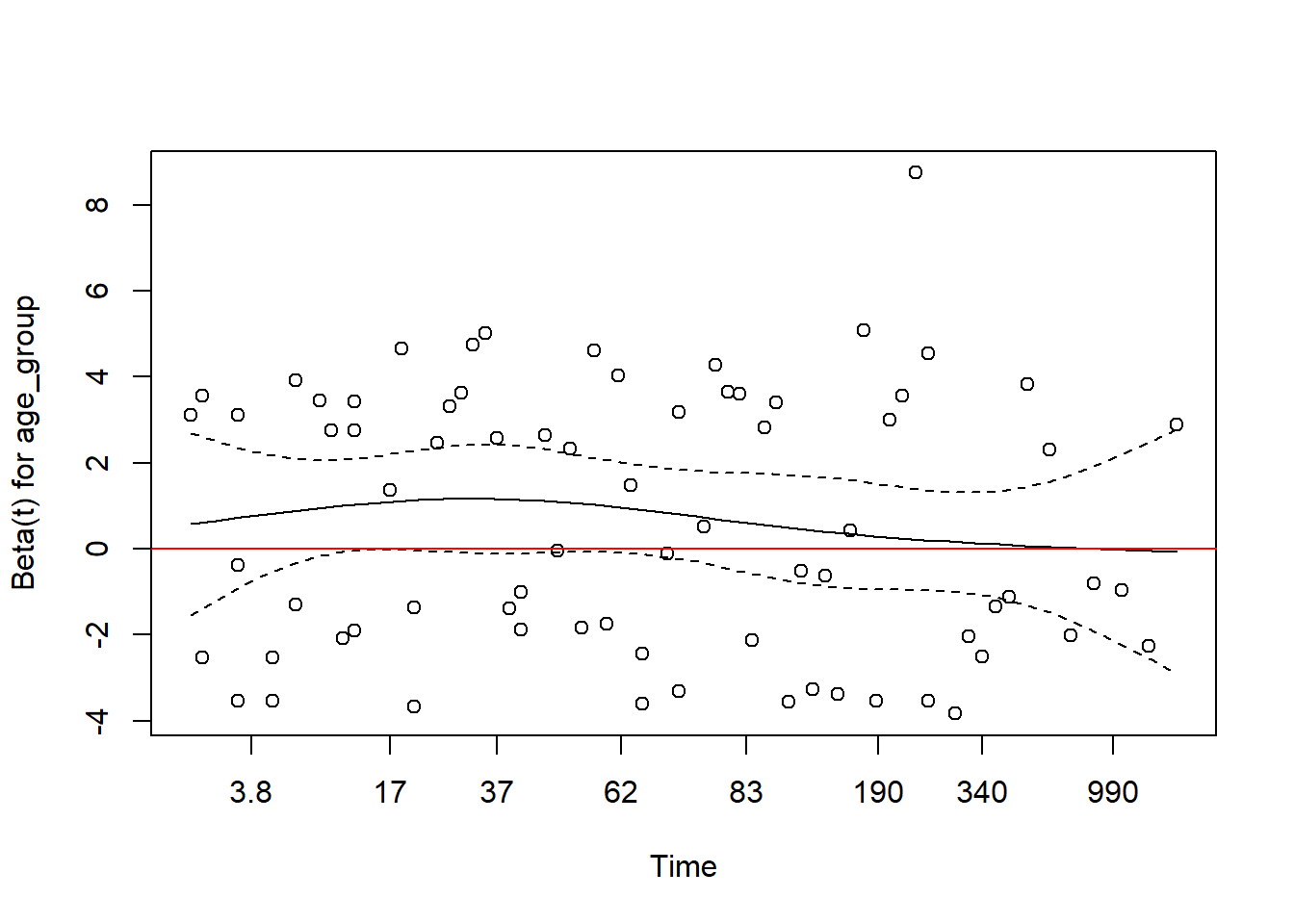plot(cox.zph(cox.md))
abline(h=0,col=2)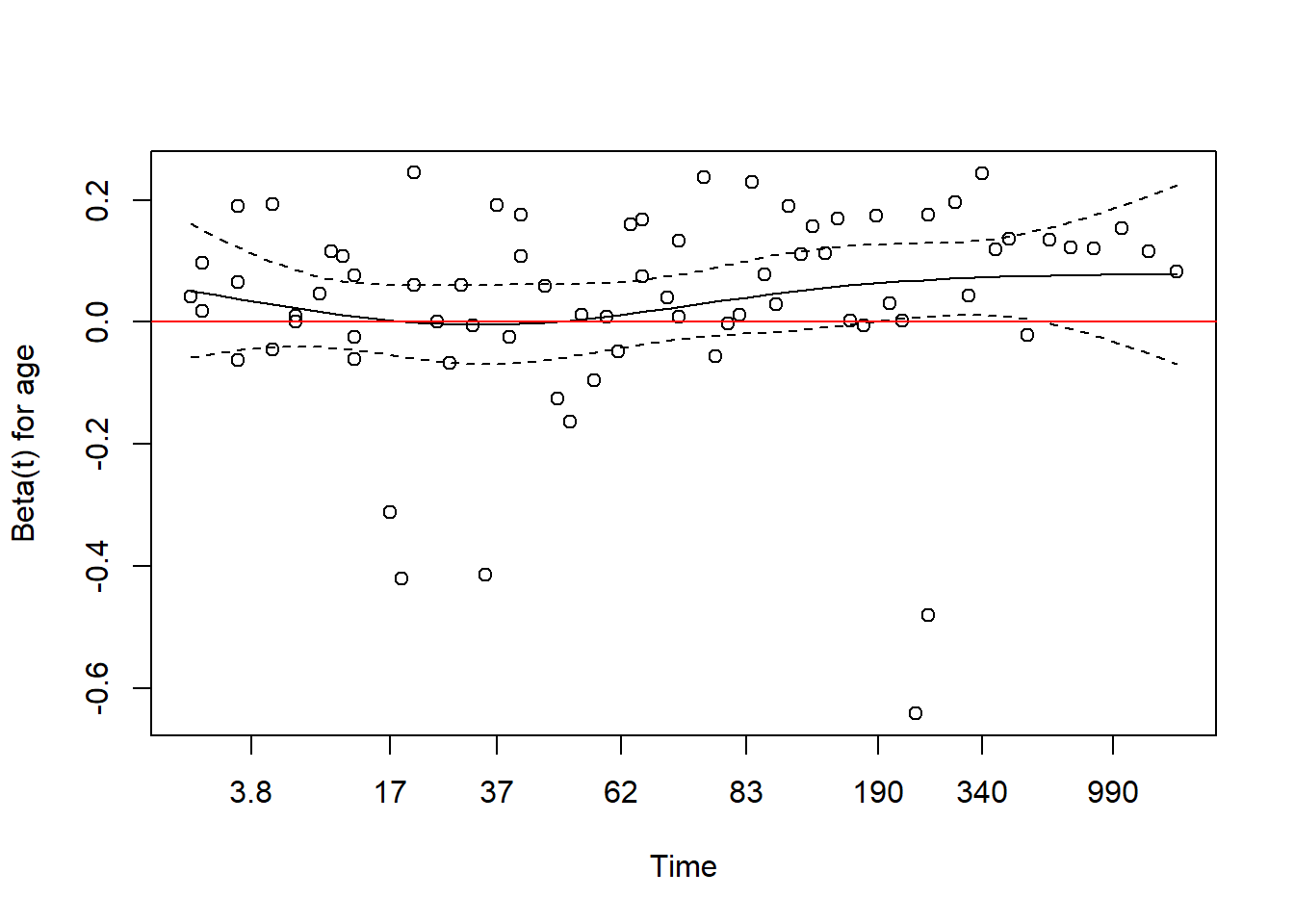plot(cox.zph(cox.md))
abline(h=0,col=2)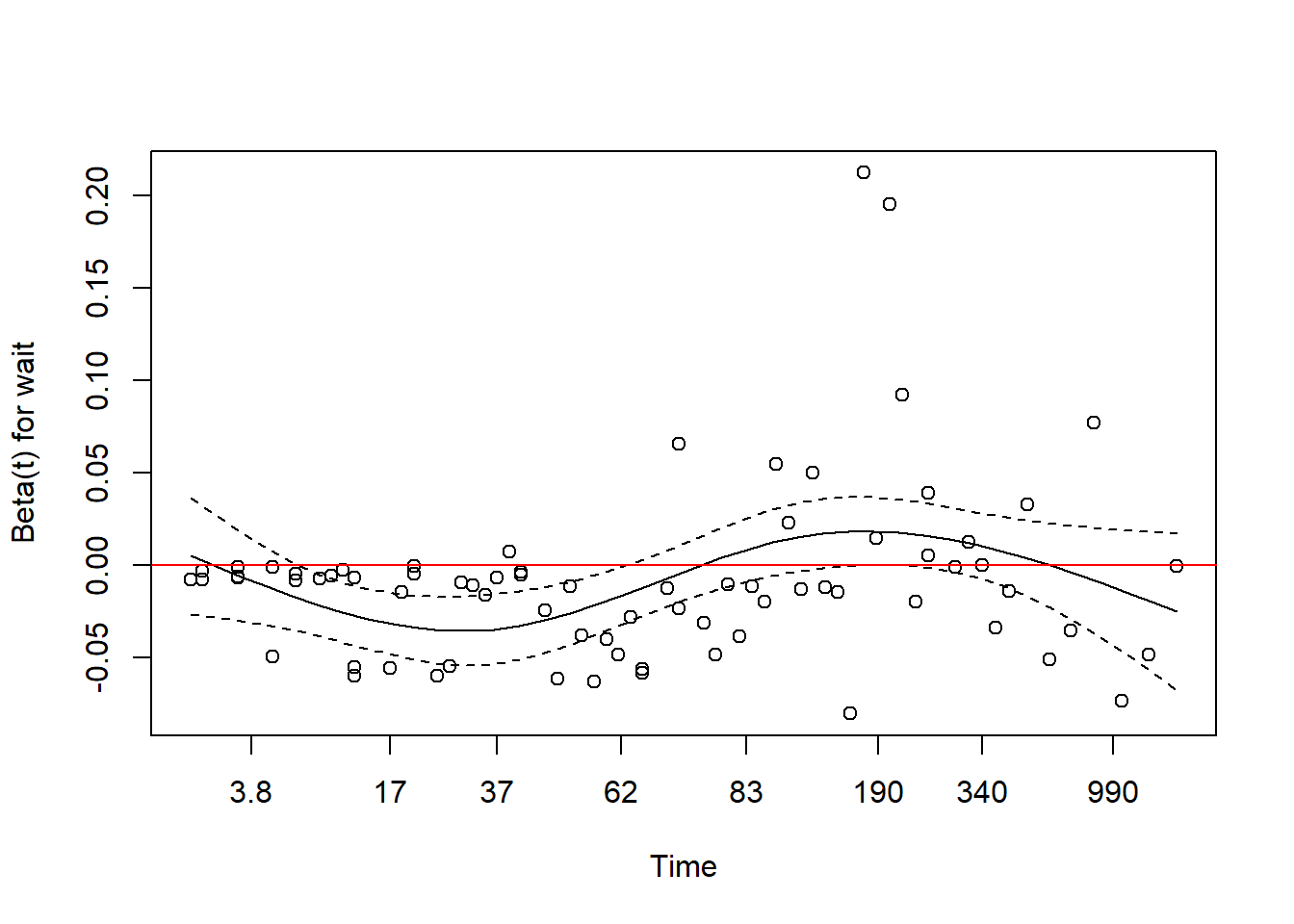### Log(-Log(S(t))) Vs Log(Time) Plots

#### Observation

Both the log-log plots, the survival model with independent variable age and survival model with the independent variable wait, clearly indicate that these models violate the proportional hazard assumption. Therefore, independent variables age and wait will not be included in the final survival model.

library(survival)
md <- survfit(Surv(survtime,survived) ~ prior, data = input_data)
plot(md, col=c("blue", "red"), fun="cloglog", xlab="Log(Time)", ylab="Log(-Log(S(t)))")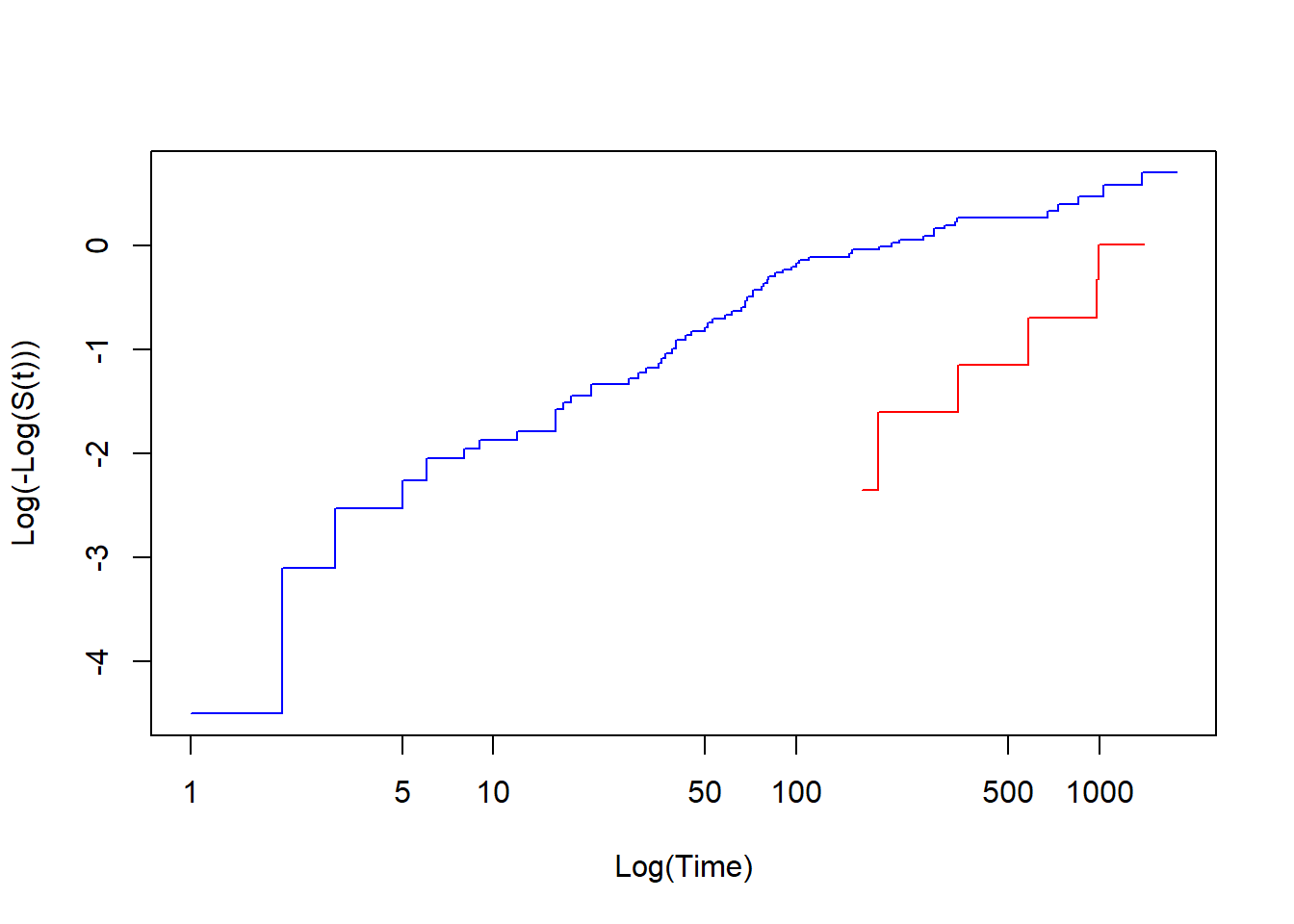library(survival)
md <- survfit(Surv(survtime,survived) ~ transplant, data = input_data)
plot(md, col=c("blue", "red"), fun="cloglog", xlab="Log(Time)", ylab="Log(-Log(S(t)))")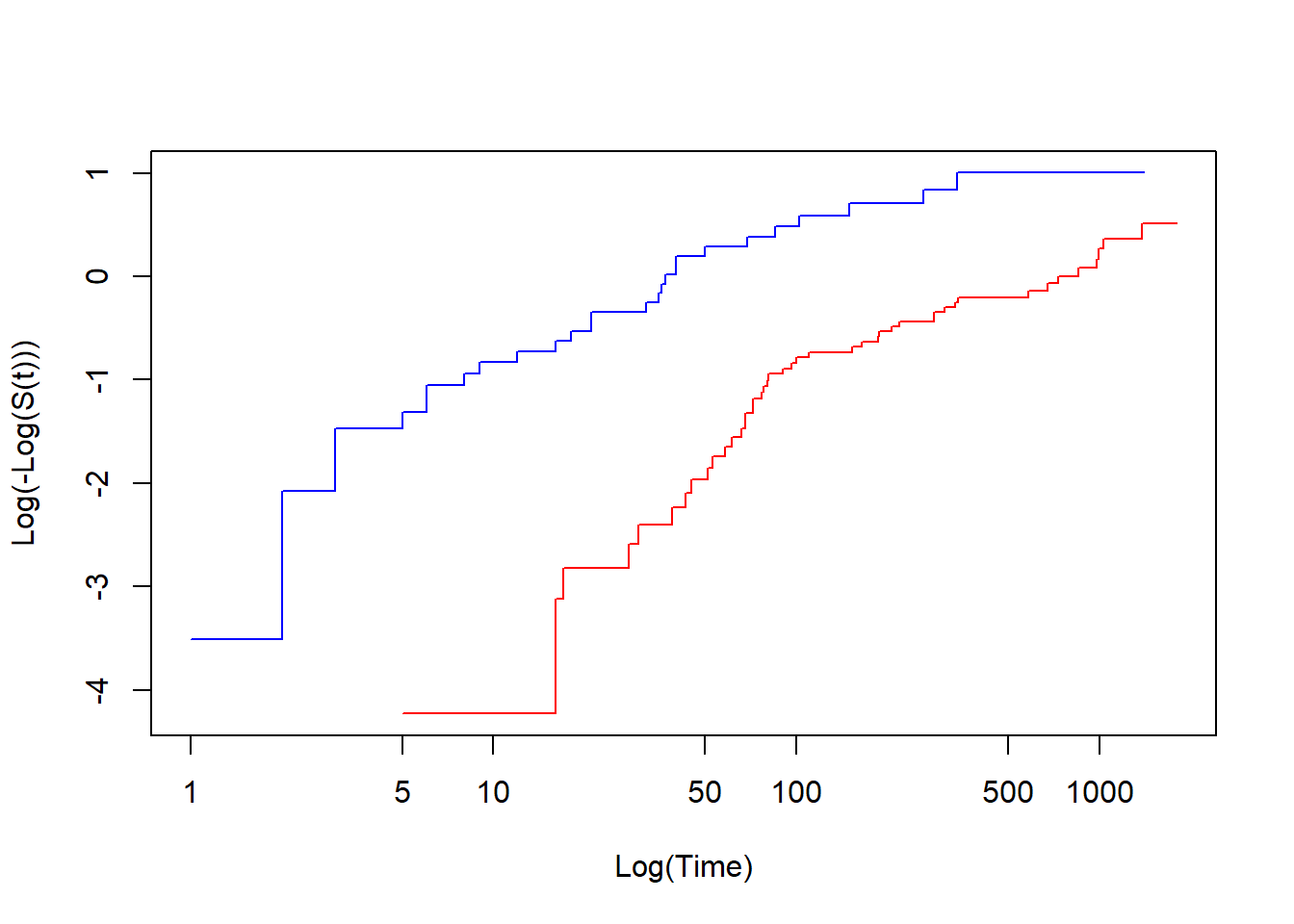library(survival)
md <- survfit(Surv(survtime,survived) ~ age_group, data = input_data)
plot(md, col=c("blue", "red"), fun="cloglog", xlab="Log(Time)", ylab="Log(-Log(S(t)))")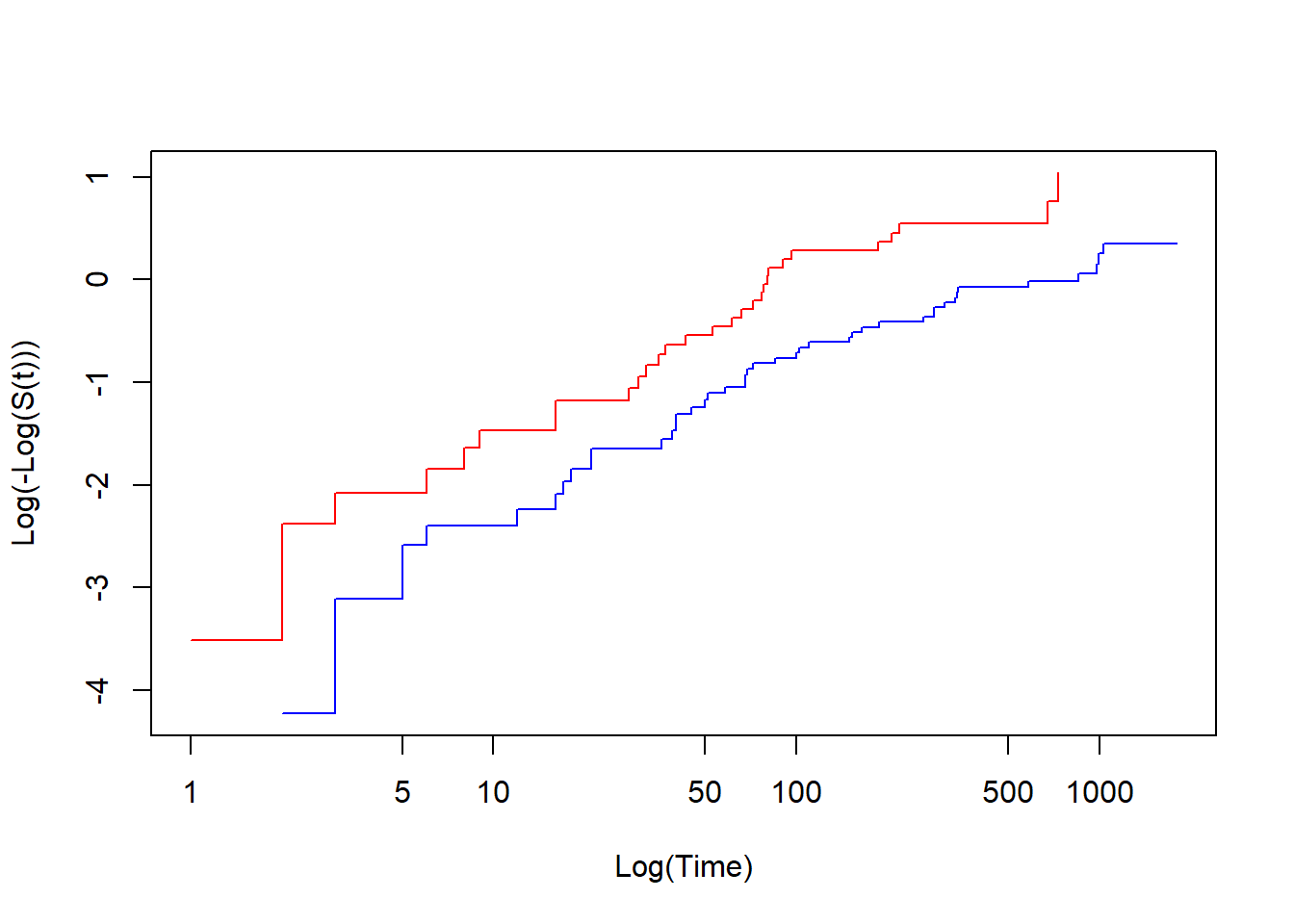library(survival)
md <- survfit(Surv(survtime,survived) ~ age, data = input_data)
plot(md, col=c("blue", "red"), fun="cloglog", xlab="Log(Time)", ylab="Log(-Log(S(t)))")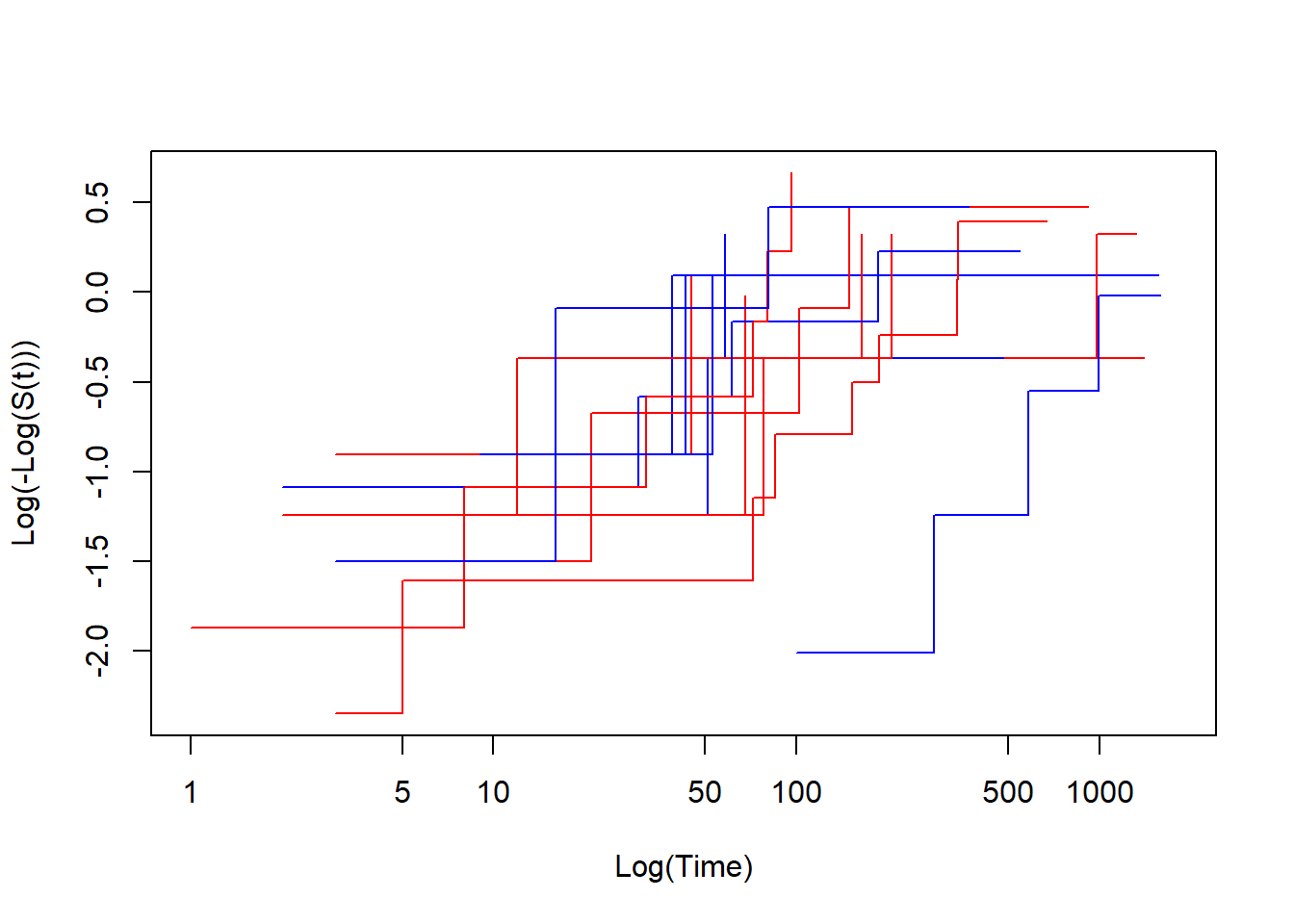library(survival)
md <- survfit(Surv(survtime,survived) ~ wait, data = input_data)
plot(md, col=c("blue", "red"), fun="cloglog", xlab="Log(Time)", ylab="Log(-Log(S(t)))")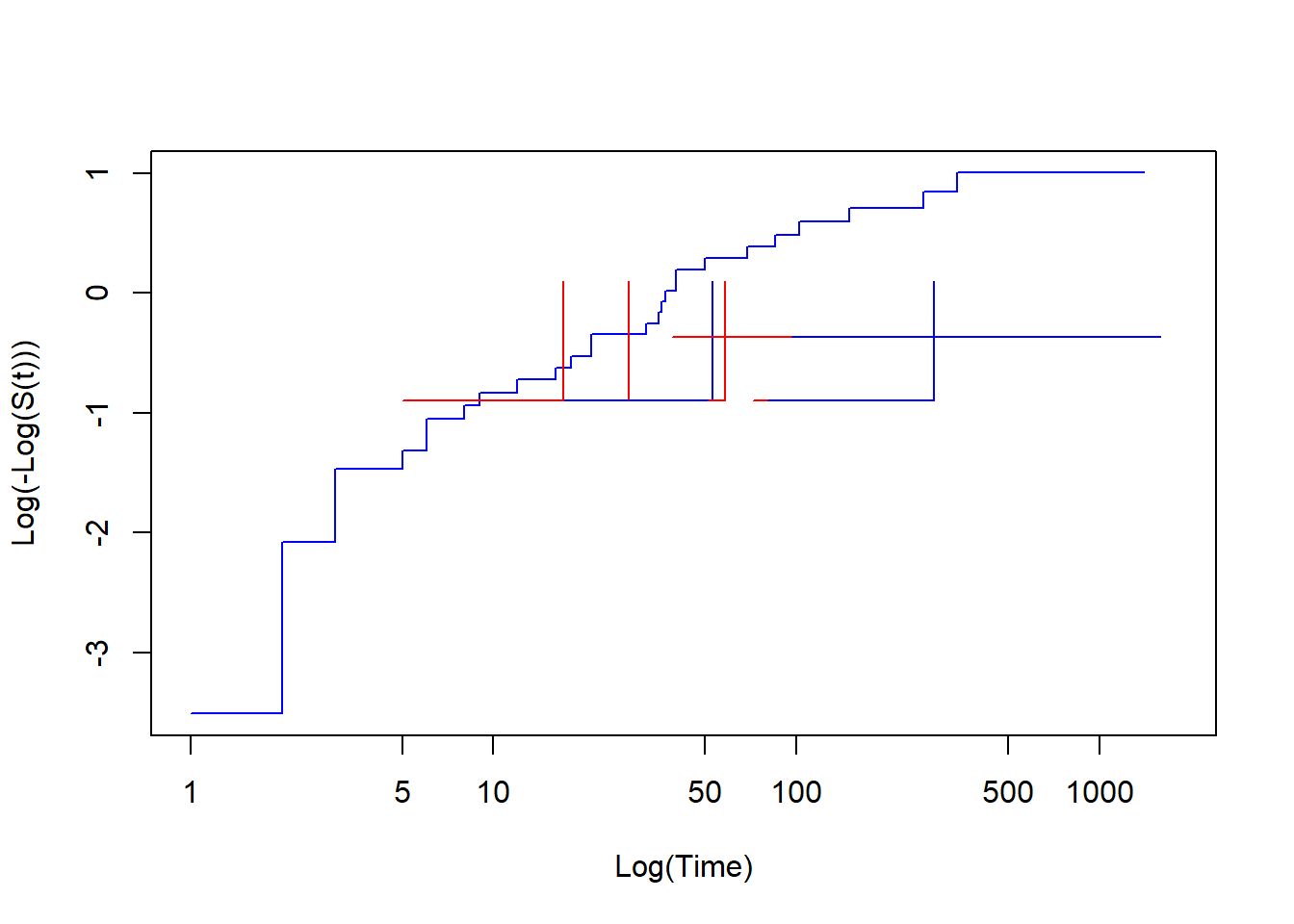### Comparing Nested Models Using Likelihood Test

#### Observation

The p-value of 0.2278 from the ANOVA comparison between the two models indicates that the difference between the two models is not significant. It is also revealed that the independent variable prior is not statistically significant. Therefore, the independent variable prior could be dropped without losing any predictive power.

#Model 1
cox.md1 <- coxph(Surv(survtime,survived) ~ prior+transplant+age_group, data = input_data)
summary(cox.md1)
## Call:
## coxph(formula = Surv(survtime, survived) ~ prior + transplant +
##     age_group, data = input_data)
##
##   n= 103, number of events= 75
##
##               coef exp(coef) se(coef)      z Pr(>|z|)
## prior      -0.5067    0.6025   0.4449 -1.139    0.255
## transplant -1.5550    0.2112   0.2738 -5.679 1.35e-08 ***
## age_group1  1.1199    3.0644   0.2595  4.315 1.59e-05 ***
## ---
## Signif. codes:  0 '***' 0.001 '**' 0.01 '*' 0.05 '.' 0.1 ' ' 1
##
##            exp(coef) exp(-coef) lower .95 upper .95
## prior         0.6025     1.6598    0.2519    1.4409
## transplant    0.2112     4.7352    0.1235    0.3612
## age_group1    3.0644     0.3263    1.8427    5.0962
##
## Concordance= 0.74  (se = 0.028 )
## Likelihood ratio test= 46.87  on 3 df,   p=4e-10
## Wald test            = 45.14  on 3 df,   p=9e-10
## Score (logrank) test = 52.67  on 3 df,   p=2e-11
#Model 2
cox.md2 <- coxph(Surv(survtime,survived) ~ transplant+age_group, data = input_data)
summary(cox.md2)
## Call:
## coxph(formula = Surv(survtime, survived) ~ transplant + age_group,
##     data = input_data)
##
##   n= 103, number of events= 75
##
##               coef exp(coef) se(coef)      z Pr(>|z|)
## transplant -1.6505    0.1920   0.2652 -6.224 4.84e-10 ***
## age_group1  1.1663    3.2101   0.2569  4.540 5.62e-06 ***
## ---
## Signif. codes:  0 '***' 0.001 '**' 0.01 '*' 0.05 '.' 0.1 ' ' 1
##
##            exp(coef) exp(-coef) lower .95 upper .95
## transplant     0.192     5.2095    0.1142    0.3228
## age_group1     3.210     0.3115    1.9403    5.3109
##
## Concordance= 0.725  (se = 0.029 )
## Likelihood ratio test= 45.41  on 2 df,   p=1e-10
## Wald test            = 44.95  on 2 df,   p=2e-10
## Score (logrank) test = 51.93  on 2 df,   p=5e-12
anova(cox.md1,cox.md2,test="LRT")
## Analysis of Deviance Table
##  Cox model: response is  Surv(survtime, survived)
##  Model 1: ~ prior + transplant + age_group
##  Model 2: ~ transplant + age_group
##    loglik  Chisq Df P(>|Chi|)
## 1 -274.69
## 2 -275.41 1.4545  1    0.2278

### Final Survival Model

cox.md <- coxph(Surv(survtime,survived) ~ transplant+age_group, data = input_data)
summary(cox.md)
## Call:
## coxph(formula = Surv(survtime, survived) ~ transplant + age_group,
##     data = input_data)
##
##   n= 103, number of events= 75
##
##               coef exp(coef) se(coef)      z Pr(>|z|)
## transplant -1.6505    0.1920   0.2652 -6.224 4.84e-10 ***
## age_group1  1.1663    3.2101   0.2569  4.540 5.62e-06 ***
## ---
## Signif. codes:  0 '***' 0.001 '**' 0.01 '*' 0.05 '.' 0.1 ' ' 1
##
##            exp(coef) exp(-coef) lower .95 upper .95
## transplant     0.192     5.2095    0.1142    0.3228
## age_group1     3.210     0.3115    1.9403    5.3109
##
## Concordance= 0.725  (se = 0.029 )
## Likelihood ratio test= 45.41  on 2 df,   p=1e-10
## Wald test            = 44.95  on 2 df,   p=2e-10
## Score (logrank) test = 51.93  on 2 df,   p=5e-12

#### Equation

ln(H(t|X)) = ln(Ho(t)) + (B1(X1) + B2(X2) = B1(transplant) + B2(age_group1))
ln(H(t|X)) = ln(Ho(t)) + (- 1.6505(transplant) + 1.1663(age_group1))
ln(Ho(t)) = Baseline hazard and represents the hazard when all of the predictor X’s are equal to zero
H(t|X) = H(t|X=0)/H(t|X=1) ~ e^(B1(X1)+B2(X2))
H(X=0) = (deads)/(number of patients in group X=0) for survival duration t
H(X=1) = (deads)/(number of patients in group X=1) for survival duration t
Survial Probability % ~ H(t|X) - 1
X1 = transplant = Flag indicates whether the patient had a heart transplant
X2 = age_group1 = Flag indicates whether the patient was younger than 50 years old
B1 = Coefficient 1 = -1.6505
B2 = Coefficient 2 = 1.1663

#### Interpretation

##### The Extreme Outcomes

X1=1 and X2=0:
If the value of dummy variable age_group1 is 0, then a value of 1 in the variable transplant corresponds to a decrease of e^-1.6505 -1 = -0.808046092 or 81% in the hazard ratio. In other words, if the patient who was younger than 50 years old had a heart transplant, his or her probability of dying will be reduced by 81%.

X1=0 and X2=1:
If the value of the variable transplant is 0, then a value of 1 in the dummy variable age_group1 corresponds to an increase of e^-1.1663-1 = 2.210093293 or 221% in the hazard ratio. In other words, the probability that a patient who was equal to or over 50 years old absolutely dying will be more than doubled if he or she did not receive the heart transplant.

##### The Normal Outcomes

X1=1 and X1=0:
If the value of dummy variable age_group1 is 1, then a value of 1 in the variable transplant corresponds to a decrease of e^(1.1663-1.6505) -1 = -0.383810048 or 38.4% in the hazard ratio. In other words, if the patient who was equal to or over 50 years old had a heart transplant, his or her probability of dying will be reduced by 38.4%.